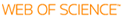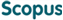### BROWSE

#### Related ResearcherVigneron, Antoine
Geometric Algorithms Lab
Research Interests
• Computational geometry, algorithms design and analysis, computational complexity

Computing the Gromov hyperbolicity of a discrete metric space

Cited 0 times inCited 0 times inTitle
Computing the Gromov hyperbolicity of a discrete metric space
Author
Issue Date
2015-07
Publisher
ELSEVIER SCIENCE BV
Citation
INFORMATION PROCESSING LETTERS, v.115, no.6-8, pp.576 - 579
Abstract
We give exact and approximation algorithms for computing the Gromov hyperbolicity of an n-point discrete metric space. We observe that computing the Gromov hyperbolicity from a fixed base-point reduces to a (max,min) matrix product. Hence, using the (max,min) matrix product algorithm by Duan and Pettie, the fixed base-point hyperbolicity can be determined in O(n(2.69)) time. It follows that the Gromov hyperbolicity can be computed in O(n(3.69)) time, and a 2-approximation can be found in O(n(2.69)) time. We also give a (2 log(2)n)-approximation algorithm that runs in O(n(2)) time, based on a tree-metric embedding by Gromov. We also show that hyperbolicity at a fixed base-point cannot be computed in O(n(2.05)) time, unless there exists a faster algorithm for (max,min) matrix multiplication than currently known.
URI
https://scholarworks.unist.ac.kr/handle/201301/19503
URL
http://www.sciencedirect.com/science/article/pii/S0020019015000198
DOI
10.1016/j.ipl.2015.02.002
ISSN
0020-0190
Appears in Collections:
CSE_Journal Papers
Files in This Item:
There are no files associated with this item.